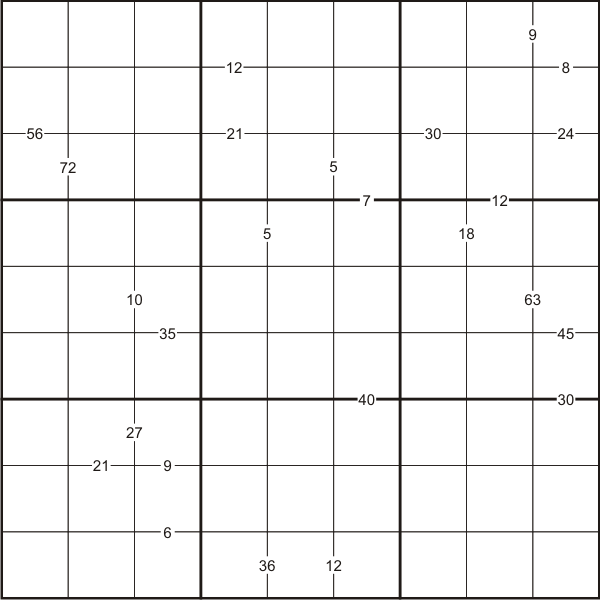#### You may also like### Exploring Wild & Wonderful Number Patterns

EWWNP means Exploring Wild and Wonderful Number Patterns Created by Yourself! Investigate what happens if we create number patterns using some simple rules.### Sending Cards

This challenge asks you to investigate the total number of cards that would be sent if four children send one to all three others. How many would be sent if there were five children? Six?### Dice and Spinner Numbers

If you had any number of ordinary dice, what are the possible ways of making their totals 6? What would the product of the dice be each time?

# A First Product Sudoku

##### Age 7 to 14Challenge LevelLike the standard Sudoku, this Sudoku consists of a grid of nine rows and nine columns subdivided into nine 3x3 subgrids.

Like the standard Sudoku, it has two basic rules:
1. Each column, each row and each box (3x3 subgrid) must have the numbers 1 to 9
2. No column, row or box can have two squares with the same number.

The puzzle can be solved with the help of clue-numbers which are small numbers written on the intersections between two cells. Each clue-number is the product of the two digits in the two cells that are on either side of it.

For example, 8 in the top right box means that possible pairs of numbers for the cells above and below are either 1 and 8, 8 and 1, 4 and 2, or 2 and 4 respectively.

You may find it useful to print out a copy of the First Product Sudoku